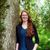# QlikView App Dev

Discussion Board for collaboration related to QlikView App Development.

Announcements
Customer & Partners, DEC. 9, 11 AM ET: Qlik Product & Strategy Roadmap Session: Data Analytics REGISTER NOW
cancel
Showing results for
Did you mean:Not applicable

## Linest & Set Analysis

Hi all,

I have a problem with generating a custom trendline (based on linest m & linest b functions) and combining it with a set analysis.

I have 12 monthly figures representing sales, through Sept 2012 - Aug 2013. Here a prerequisite is that when I choose one month the previous 11 months + the currently selected month is visible in the chart. For this I inject a set analysis like:

( { <Year =, Month ={">=\$(='201209') <=\$(=max(Month))"}>} Sales)                              (nevermind the hardcoding of the monthnum)

So now I want to create a trendline which only plots the trend for the sales in 2013. I figured I inject the same set expression into the linest expression as follows:

( { <Year =, Month ={">=\$(='201301') <=\$(=max(Month))"}>} total aggr(sum( { <Year =, Month ={">=\$(='201301') <=\$(=max(Month))"}>}  Sales),Month),Month)*Month
+
linest_b( { <Year =, Month ={">=\$(='201301') <=\$(=max(Month))"}>} total aggr(sum( { <Year =, Month ={">=\$(='201301') <=\$(=max(Month))"}>}  Sales),Month),Month)

However this only returns a dot.

I have experimented without the set analysis, in fact selecting the entire period. And it Works fine with the manual linest trendline, however it is a requirement that the set expression is included, due to the fact that I want to choose only one month and get the rolling 12M figures displayed in the chart.

Please see my attached example, for further understanding. In the file a "QV generated" trendline is plotted for the Whole series (blue line). And the manual trendline that I am trying to create is visible as a red dot.

Any ideas on how to solve this problem??

Thank you,

David

1 Solution

Accepted SolutionsMVP

Try

linest_m( { <Year =, Month ={">=201301<=\$(=max(Month))"}>} total aggr( sum( { <Year =, Month ={">=201301<=\$(=max(Month))"}>}  Sales),Month),Month )

*only({ <Year =, Month ={">=201301<=\$(=max(Month))"}>}Month)

+linest_b( { <Year =, Month ={">=201301<=\$(=max(Month))"}>} total aggr(sum( { <Year =, Month ={">=201301<=\$(=max(Month))"}>}  Sales),Month),Month )

5 RepliesMVP

linest_m(total aggr(sum( { <Year =, Month ={">=\$(='201301') <=\$(=max(Month))"}>}  Sales),Month),Month)*Month

+linest_b(total aggr(sum( { <Year =, Month ={">=\$(='201301') <=\$(=max(Month))"}>}  Sales),Month),Month)MVP

Try

linest_m( { <Year =, Month ={">=201301<=\$(=max(Month))"}>} total aggr( sum( { <Year =, Month ={">=201301<=\$(=max(Month))"}>}  Sales),Month),Month )

*only({ <Year =, Month ={">=201301<=\$(=max(Month))"}>}Month)

+linest_b( { <Year =, Month ={">=201301<=\$(=max(Month))"}>} total aggr(sum( { <Year =, Month ={">=201301<=\$(=max(Month))"}>}  Sales),Month),Month )Not applicable
Author

Thanks swuel!

Indeed this is what I was looking for!Not applicable
Author